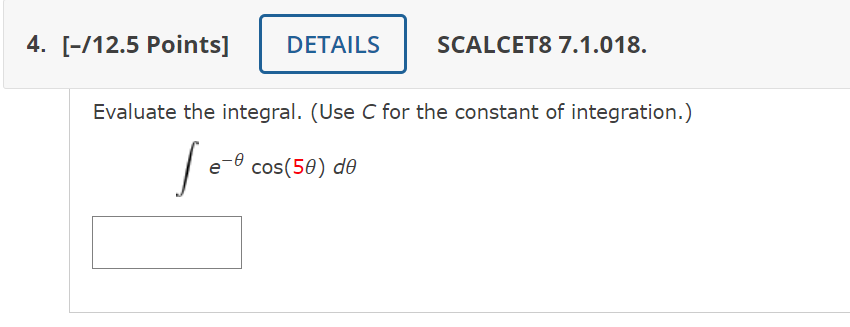Home / Expert Answers / Calculus / evaluate-the-integral-use-c-for-the-constant-of-integration-int-e-theta-cos-5-theta-pa974

# (Solved): Evaluate the integral. (Use C for the constant of integration.) $\int e^{-\theta} \cos (5 \theta ...Evaluate the integral. (Use C for the constant of integration.) \[ \int e^{-\theta} \cos (5 \theta) d \theta$

We have an Answer from Expert